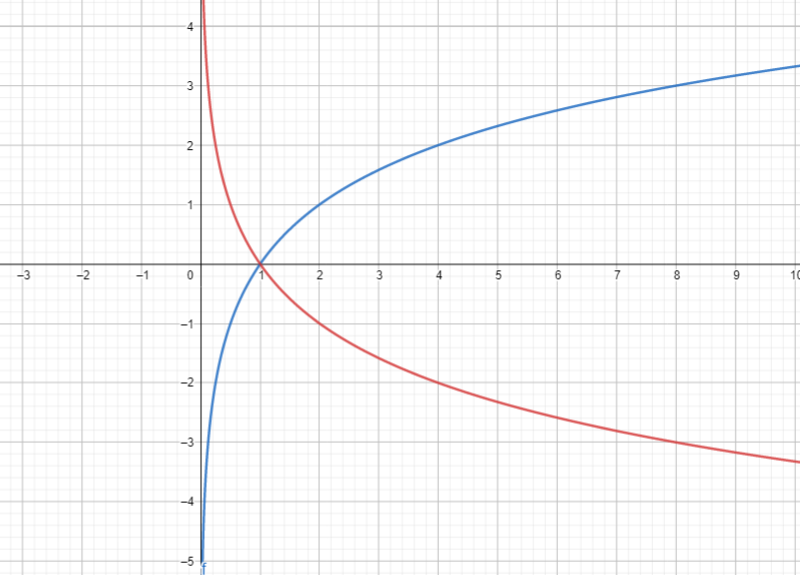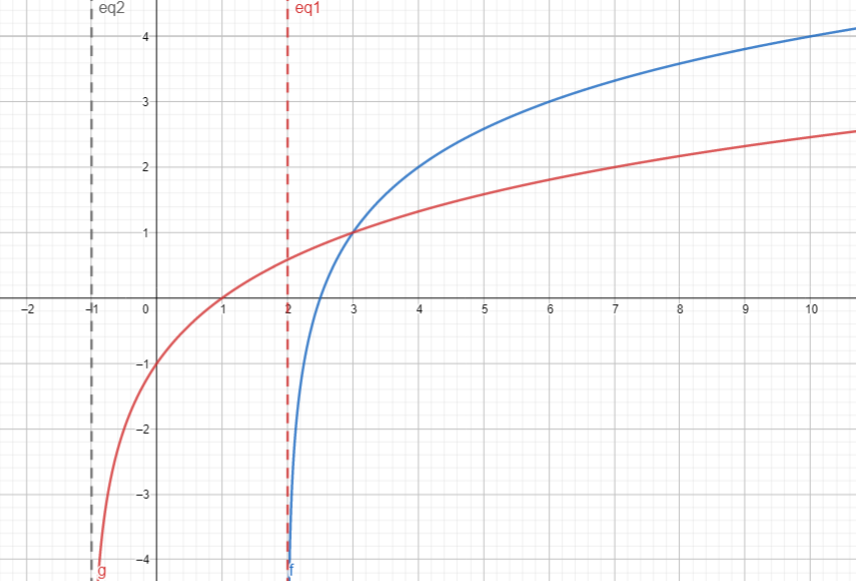407 493 6601

# Algebra 2 - Logarithms

## Introduction - Quick Review on Laws of Logarithms and Properties

• A logarithm is another term to denote an exponent.
• The logarithm of a positive number $y$ to a base $b$ (where $b$ is not equal to 1) is the exponent of $x$ to which the base must be raised to obtain the number $y$. Hence, if  this is written as $y={b}^{x}$ and the inverse is in logarithmic form $y={\mathrm{log}}_{b}\left(x\right)$.
• The expression ${\mathrm{log}}_{b}\left(x\right)$is read as logarithm $x$ to the base $b$.

Key Points in Logarithms:

• There are two types of logarithms - common logarithms and natural logarithms. Common logarithms are logarithms whose base is 10. In symbols, we have: . Natural logarithms are logarithms whose base is an irrational number $e$. In symbols, we have:
• The logarithm of a negative number is not used in numerical computations.
• A logarithm of 0 does not exist.
• Numbers between 0 and 1 have negative logarithms.
• The logarithm of 1 is 0.
• The logarithm of any number greater than 1 is always positive.

Laws of Logarithms:

Assume that  are greater than zero and not equal to 1.

• Product Rule: ${\mathrm{log}}_{b}\left(XY\right)={\mathrm{log}}_{b}\left(X\right)+{\mathrm{log}}_{b}\left(Y\right)$
• Quotient Rule: ${\mathrm{log}}_{b}\left(\frac{X}{Y}\right)={\mathrm{log}}_{b}\left(X\right)-{\mathrm{log}}_{b}\left(Y\right)$
• Power Rule: ${\mathrm{log}}_{b}\left({X}^{r}\right)=r{\mathrm{log}}_{b}\left(X\right)$
• ${\mathrm{log}}_{b}\left(\frac{1}{X}\right)=-{\mathrm{log}}_{b}\left(X\right)$
• ${b}^{{\mathrm{log}}_{b}\left(x\right)}=x$
• ${\mathrm{log}}_{b}\left({b}^{x}\right)=x$
• One-to-One Property: If  or If

Changing exponential equations to logarithmic form or vice versa:

• If ${15}^{2}=225$, then its logarithmic form is ${\mathrm{log}}_{15}\left(225\right)=2$.
• If ${\mathrm{log}}_{2}\left(\frac{1}{8}\right)=-3$, then its exponential form is ${2}^{-3}=\frac{1}{8}$

The Change of Base Formula:

Let  be positive real numbers such that . The expression can be converted to a different base like .

Laws of Logarithms are typically used to condense or expand logarithmic expressions.

Example 1. Write the expression ${\mathrm{log}}_{n}\left(2x+1\right)+{\mathrm{log}}_{n}\left(x-1\right)$ as a single logarithm.

Solution: Use the Product Rule.

Answer: ${\mathrm{log}}_{n}\left(2x+1\right)\left(x+1\right)$

Example 2. Write the expression ${\mathrm{log}}_{b}\left(x+1\right)+{\mathrm{log}}_{b}\left(3\right)-{\mathrm{log}}_{b}\left(x+2\right)$ as a single logarithm.

Solution: Use both Product Rule and Quotient Rule.

Answer: ${\mathrm{log}}_{b}\left(\frac{\left(x+1\right)\left(3\right)}{x+2}\right)$

Example 3. Write the expression ${\mathrm{log}}_{5}\left({x}^{4}{y}^{2}\right)$ in expanded form.

Solution: Use both Product Rule and Power Rule.

Answer: $4{\mathrm{log}}_{5}\left(x\right)+2{\mathrm{log}}_{5}\left(y\right)$

Example 4. Change ${\mathrm{log}}_{49}\left(2401\right)$ using base 7.

Solution:

${\mathrm{log}}_{49}\left(2401\right)=\frac{{\mathrm{log}}_{7}\left(2401\right)}{{\mathrm{log}}_{7}\left(49\right)}$

## Logarithmic Functions and Logarithmic Equations

• Logarithmic Equation is an equation involving logarithmic expressions. This relation may involve a variable on the base, a variable on the logarithm, and either one side of the equation contains a logarithmic expression, or both sides involve logarithmic expressions.
• Some examples of logarithmic equations are:
• Logarithmic Function with base $b$ where , is the inverse of the exponential function with base $b$
• The logarithmic function is denoted by $f\left(x\right)={\mathrm{log}}_{b}\left(x\right)$ if and only if $x={b}^{y}$ for every $x>0$ and every real number $y.$
• Based on the definition, the following are examples of logarithmic function are:

## Solving Logarithmic Equations

• To solve for the value of the unknown in logarithmic equations, we need to apply the one-to-one property and laws of logarithms.
• One-to-one property: "If $X=Y$, then " or "If .

Step in solving logarithmic equations:

• Set aside the logarithmic expression on one side of the equation.
• Combination of like logarithmic expressions is suggested by using the appropriate laws of the logarithm.
• Express the logarithmic equation in exponential form.
• Solve for the value of the unknown.

Example 1. Find the solution of ${\mathrm{log}}_{2}\left(5x+3\right)-{\mathrm{log}}_{2}\left(4x-3\right)=3$.

Solution: Use Quotient Rule to condense the expression.

${\mathrm{log}}_{2}\left(5x+3\right)-{\mathrm{log}}_{2}\left(4x-3\right)=3\to {\mathrm{log}}_{2}\left(\frac{5x+3}{4x-3}\right)=3$

Express ${\mathrm{log}}_{2}\left(\frac{5x+3}{4x-3}\right)=3$ in exponential form: $\frac{5x+3}{4x-3}={2}^{3}\to 5x+3=8\left(4x-3\right)\to \mathbit{x}\mathbf{=}\mathbf{1}$

Example 2. What is/are the solution(s) of ${\mathrm{log}}_{3}\left(x+2\right)+{\mathrm{log}}_{3}\left(3x-4\right)={\mathrm{log}}_{3}\left(3x+2\right)$?

Solution: Use the Product Rule to condense the expression.

${\mathrm{log}}_{3}\left(x+2\right)+{\mathrm{log}}_{3}\left(3x-4\right)={\mathrm{log}}_{3}\left(3x+2\right)\to {\mathrm{log}}_{3}\left(x+2\right)\left(3x-4\right)={\mathrm{log}}_{3}\left(3x+2\right)$

Use the one-to-one property to remove the logarithm in ${\mathrm{log}}_{3}\left(x+2\right)\left(3x-4\right)={\mathrm{log}}_{3}\left(3x+2\right)$${\mathrm{log}}_{3}\left(x+2\right)\left(3x-4\right)={\mathrm{log}}_{3}\left(3x+2\right)\to \left(x+2\right)\left(3x-4\right)=3x+2$

This forms a quadratic equation $3{x}^{2}-x-10=0$ that has roots .

The only acceptable solution is $\mathbit{x}\mathbf{=}\mathbf{2}\mathbf{.}$

## Properties and Graphs of Logarithmic Functions

• The domain of logarithmic functions is the set of all positive real numbers. The Domain of the logarithmic function = Range of the exponential function.
• The range consists of all real numbers. The Range of the logarithmic function = Domain of the exponential function.
• The function increases (the curve is rising) for $b>1$ and decreases for $0.
• It is considered a one-to-one function.
• For cases like $y={\mathrm{log}}_{b}\left(x\right)$, the x-intercept is 1. There is no y-intercept except for cases like $y=a{\mathrm{log}}_{b}\left(x-c\right)+d$.
• For cases like $y={\mathrm{log}}_{b}\left(x\right)$, the vertical asymptote is $x=0.$

1. Study the graphs below:Graph of $y={\mathrm{log}}_{2}\left(x\right)$ (blue curve) and $y={\mathrm{log}}_{\frac{1}{2}}\left(x\right)$ (red curve)

2. Study the graphs below:Graph of $y={\mathrm{log}}_{2}\left(x-2\right)+1$ (blue curve) and $y={\mathrm{log}}_{2}\left(x+1\right)-1$ (red curve). Notice their vertical asymptotes.

For the case of $y=a{\mathrm{log}}_{b}\left(x-c\right)+d$:

• The value of $b$, either  determines whether the graph has an increasing or decreasing trend.
• The value of $a$ tells the stretching and shrinking of the graph. If $a<0$ or $a>0$, there is a reflection of the graph about the x-axis.
• Based on the parent function $y={\mathrm{log}}_{b}\left(x\right),$ the vertical shift is $d$ units upward when $d>0$ or $d$ units downward when if $d<0.$
• The horizontal shift is $c$ units to the right when $c>0$ or $c$ units to the left when $c<0.$

## Cheat Sheet

• Always keep in mind the laws and properties of logarithms when dealing with logarithmic equations and logarithmic functions.
• To find the x-intercept of a logarithmic function, set the variable $y$ to 0 and solve for $x$. When solving for the x-intercept, use the process of solving logarithmic equations.
• The vertical asymptote of the function $y={\mathrm{log}}_{\frac{1}{3}}\left(2x+3\right)-1$ is $x=-\frac{3}{2}$.
• The transformation of the graph of $y={\mathrm{log}}_{4}\left(x-3\right)-2$ is described as: The graph of $y={\mathrm{log}}_{4}\left(x\right)$ shifts three units to the right and 2 units downward.
• The laws of common logarithms and logarithms with other bases are applicable to natural logarithms.
• In natural logarithms, $\mathrm{ln}\left(e\right)=1$ and ${e}^{\mathrm{ln}\left(x\right)}=x$.

## Blunder Areas

• Exponential equations that cannot be expressed with common bases can be solved using logarithms. Some examples are .
• In solving logarithmic equations, always inspect whether the obtained roots satisfy the equation or not. In cases where the resulting equation is quadratic, one obtained solution may or may not be extraneous.
• Always establish the idea that in $y={\mathrm{log}}_{b}\left(x\right)$$x>0.$
• When two logarithmic expressions in an equation have different bases, the equation cannot be solved directly using one-to-one property.